Machine learning interview questions are an integral part of the data science interview and the path to becoming a data scientist, machine learning engineer, or data engineer. In order to help resolve that, here is a curated and created a list of key questions that you could see in a machine learning interview. There are some answers to go along with them so you don’t get stumped. You’ll be able to do well in any job interview (even for a machine learning internship) with after reading through this piece. This is second part of Top Machine learning interview questions.

Visit part 1 for more interview questions related to Machine Learning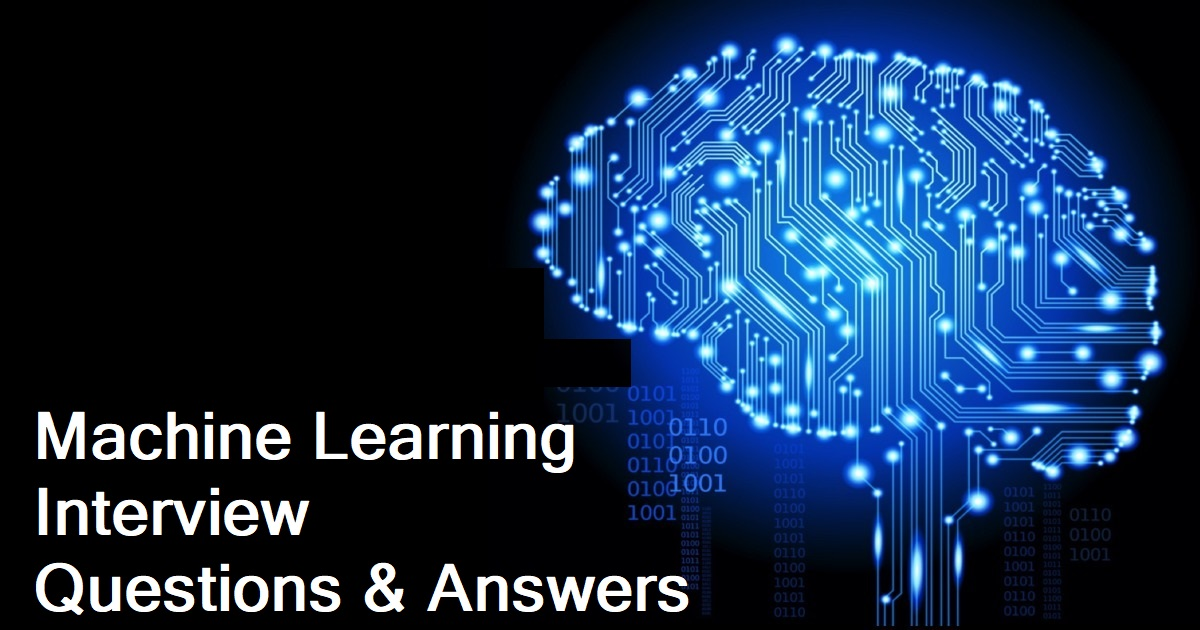36) What is the general principle of an ensemble method and what is bagging and boosting in ensemble method?

The general principle of an ensemble method is to combine the predictions of several models built with a given learning algorithm in order to improve robustness over a single model.  Bagging is a method in ensemble for improving unstable estimation or classification schemes.  While boosting method are used sequentially to reduce the bias of the combined model.  Boosting and Bagging both can reduce errors by reducing the variance term.

37) What is bias-variance decomposition of classification error in ensemble method?

The expected error of a learning algorithm can be decomposed into bias and variance. A bias term measures how closely the average classifier produced by the learning algorithm matches the target function.  The variance term measures how much the learning algorithm’s prediction fluctuates for different training sets.

38) What is an Incremental Learning algorithm in ensemble?

Incremental learning method is the ability of an algorithm to learn from new data that may be available after classifier has already been generated from already available dataset.

39) What is PCA, KPCA and ICA used for?

PCA (Principal Components Analysis), KPCA ( Kernel based Principal Component Analysis) and ICA ( Independent Component Analysis) are important feature extraction techniques used for dimensionality reduction.

40) What is dimension reduction in Machine Learning?

In Machine Learning and statistics, dimension reduction is the process of reducing the number of random variables under considerations and can be divided into feature selection and feature extraction.

41) What are support vector machines?

Support vector machines are supervised learning algorithms used for classification and regression analysis.

42) What are the components of relational evaluation techniques?

The important components of relational evaluation techniques are

• Data Acquisition
• Ground Truth Acquisition
• Cross Validation Technique
• Query Type
• Scoring Metric
• Significance Test

43) What are the different methods for Sequential Supervised Learning?

The different methods to solve Sequential Supervised Learning problems are

• Sliding-window methods
• Recurrent sliding windows
• Hidden Markow models
• Maximum entropy Markow models
• Conditional random fields
• Graph transformer networks

44) What are the areas in robotics and information processing where sequential prediction problem arises?

The areas in robotics and information processing  where sequential prediction problem arises are

• Imitation Learning
• Structured prediction
• Model based reinforcement learning

45) What is batch statistical learning?

Statistical learning techniques allow learning a function or predictor from a set of observed data that can make predictions about unseen or future data. These techniques provide guarantees on the performance of the learned predictor on the future unseen data based on a statistical assumption on the data generating process.

46)   What is PAC Learning?

PAC (Probably Approximately Correct) learning is a learning framework that has been introduced to analyze learning algorithms and their statistical efficiency.

47) What are the different categories you can categorized the sequence learning process?

• Sequence prediction
• Sequence generation
• Sequence recognition
• Sequential decision

48) What is sequence learning?

Sequence learning is a method of teaching and learning in a logical manner.

49) What are two techniques of Machine Learning?

The two techniques of Machine Learning are

• Genetic Programming
• Inductive Learning

50) Give a popular application of machine learning that you see on day to day basis?

The recommendation engine implemented by major ecommerce websites uses Machine Learning.

51) What is a Confusion Matrix?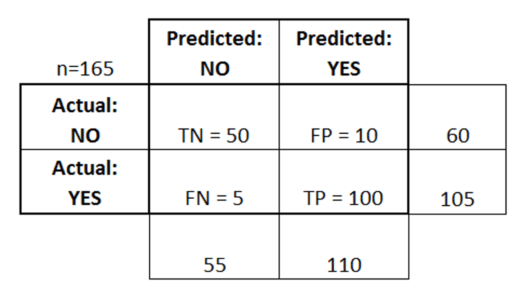A confusion matrix or an error matrix is a table which is used for summarizing the performance of a classification algorithm.

Consider the above table where:

• TN = True Negative
• TP = True Positive
• FN = False Negative
• FP = False Positive

52) What is the difference between inductive and deductive learning?

• Inductive learning is the process of using observations to draw conclusions
• Deductive learning is the process of using conclusions to form observations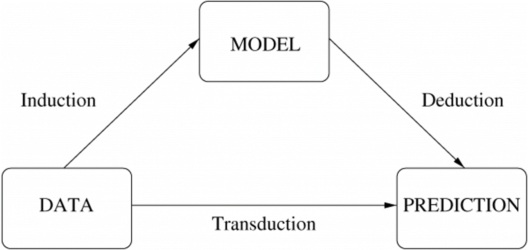53) How is KNN different from K-means clustering?54)  What is ROC curve and what does it represent?

Receiver Operating Characteristic curve (or ROC curve) is a fundamental tool for diagnostic test evaluation and is a plot of the true positive rate (Sensitivity) against the false positive rate (Specificity) for the different possible cut-off points of a diagnostic test.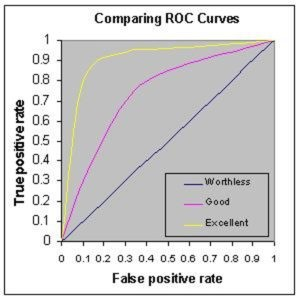• It shows the tradeoff between sensitivity and specificity (any increase in sensitivity will be accompanied by a decrease in specificity).
• The closer the curve follows the left-hand border and then the top border of the ROC space, the more accurate the test.
• The closer the curve comes to the 45-degree diagonal of the ROC space, the less accurate the test.
• The slope of the tangent line at a cutpoint gives the likelihood ratio (LR) for that value of the test.
• The area under the curve is a measure of test accuracy.

55) What’s the difference between Type I and Type II error?56) Is it better to have too many false positives or too many false negatives? Explain.It depends on the question as well as on the domain for which we are trying to solve the problem. If you’re using Machine Learning in the domain of medical testing, then a false negative is very risky, since the report will not show any health problem when a person is actually unwell. Similarly, if Machine Learning is used in spam detection, then a false positive is very risky because the algorithm may classify an important email as spam.

57) Which is more important to you – model accuracy or model performance?Well, you must know that model accuracy is only a subset of model performance. The accuracy of the model and performance of the model are directly proportional and hence better the performance of the model, more accurate are the predictions.

58) What is the difference between Gini Impurity and Entropy in a Decision Tree?

• Gini Impurity and Entropy are the metrics used for deciding how to split a Decision Tree.
• Gini measurement is the probability of a random sample being classified correctly if you randomly pick a label according to the distribution in the branch.
• Entropy is a measurement to calculate the lack of information. You calculate the Information Gain (difference in entropies) by making a split. This measure helps to reduce the uncertainty about the output label.

59)  What is the difference between Entropy and Information Gain?

• Entropy is an indicator of how messy your data is. It decreases as you reach closer to the leaf node.
• The Information Gain is based on the decrease in entropy after a dataset is split on an attribute. It keeps on increasing as you reach closer to the leaf node.

60) What is Overfitting? And how do you ensure you’re not overfitting with a model?

Over-fitting occurs when a model studies the training data to such an extent that it negatively influences the performance of the model on new data.

This means that the disturbance in the training data is recorded and learned as concepts by the model. But the problem here is that these concepts do not apply to the testing data and negatively impact the model’s ability to classify the new data, hence reducing the accuracy on the testing data.

Three main methods to avoid overfitting:

• Collect more data so that the model can be trained with varied samples.
• Use ensembling methods, such as Random Forest. It is based on the idea of bagging, which is used to reduce the variation in the predictions by combining the result of multiple Decision trees on different samples of the data set.
• Choose the right algorithm.

61) Explain Ensemble learning technique in Machine Learning.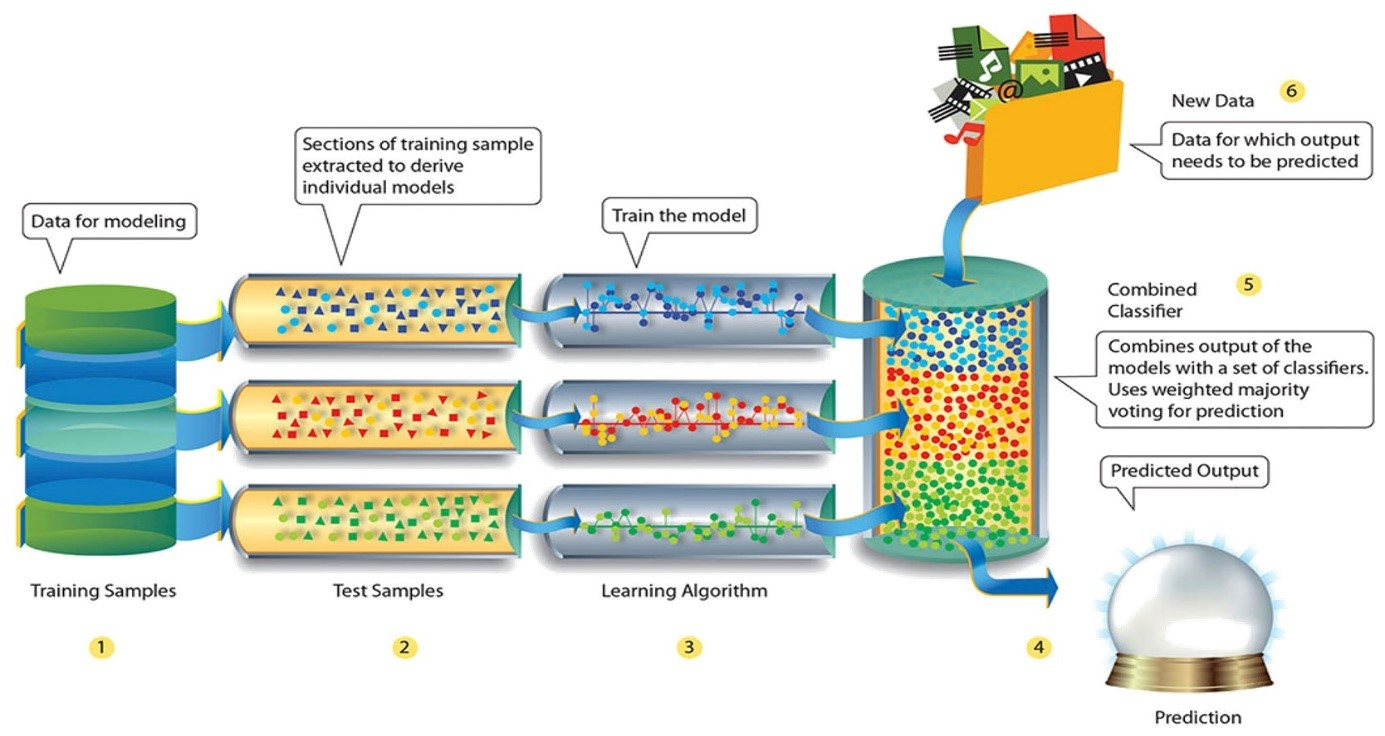Ensemble learning is a technique that is used to create multiple Machine Learning models, which are then combined to produce more accurate results. A general Machine Learning model is built by using the entire training data set. However, in Ensemble Learning the training data set is split into multiple subsets, wherein each subset is used to build a separate model. After the models are trained, they are then combined to predict an outcome in such a way that the variance in the output is reduced.

62) What is bagging and boosting in Machine Learning?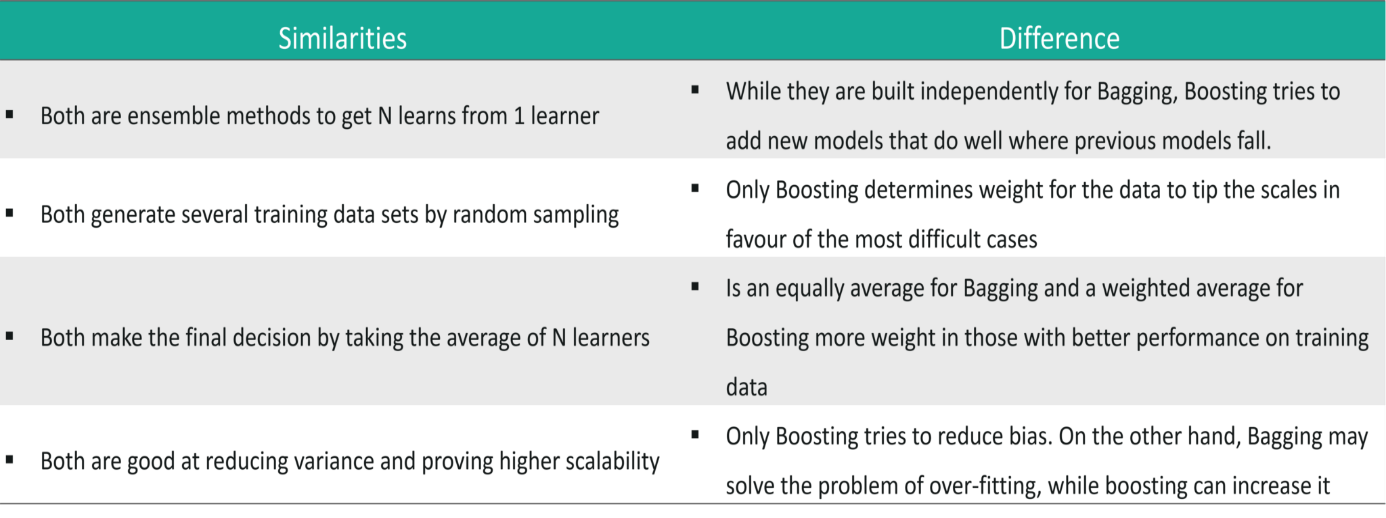63) How would you screen for outliers and what should you do if you find one?

The following methods can be used to screen outliers:

1. Boxplot: A box plot represents the distribution of the data and its variability. The box plot contains the upper and lower quartiles, so the box basically spans the Inter-Quartile Range (IQR). One of the main reasons why box plots are used is to detect outliers in the data. Since the box plot spans the IQR, it detects the data points that lie outside this range. These data points are nothing but outliers.
2. Probabilistic and statistical models: Statistical models such as normal distribution and exponential distribution can be used to detect any variations in the distribution of data points. If any data point is found outside the distribution range, it is rendered as an outlier.
3. Linear models: Linear models such as logistic regression can be trained to flag outliers. In this manner, the model picks up the next outlier it sees.
4. Proximity-based models: An example of this kind of model is the K-means clustering model wherein, data points form multiple or ‘k’ number of clusters based on features such as similarity or distance. Since similar data points form clusters, the outliers also form their own cluster. In this way, proximity-based models can easily help detect outliers.

How do you handle these outliers?

• If your data set is huge and rich then you can risk dropping the outliers.
• However, if your data set is small then you can cap the outliers, by setting a threshold percentile. For example, the data points that are above the 95th percentile can be used to cap the outliers.
• Lastly, based on the data exploration stage, you can narrow down some rules and impute the outliers based on those business rules.

64) What are collinearity and multicollinearity?

• Collinearity occurs when two predictor variables (e.g., x1 and x2) in a multiple regression have some correlation.
• Multicollinearity occurs when more than two predictor variables (e.g., x1, x2, and x3) are inter-correlated.

65) What do you understand by Eigenvectors and Eigenvalues?

• Eigenvectors: Eigenvectors are those vectors whose direction remains unchanged even when a linear transformation is performed on them.
• Eigenvalues: Eigenvalue is the scalar that is used for the transformation of an Eigenvector.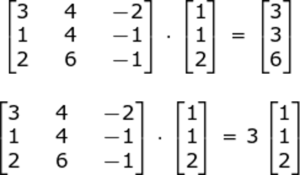In the above example, 3 is an Eigenvalue, with the original vector in the multiplication problem being an eigenvector.

Data Science Training

The Eigenvector of a square matrix A is a nonzero vector x such that for some number λ, we have the following:

Ax = λx,

where λ is an Eigenvalue
So, in our example, λ = 3 and X = [1 1 2]

66) What is A/B Testing?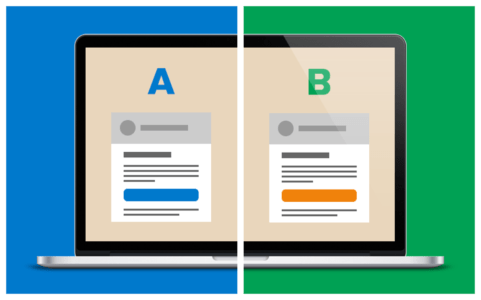###### A/B Testing
• A/B is Statistical hypothesis testing for randomized experiment with two variables A and B. It is used to compare two models that use different predictor variables in order to check which variable fits best for a given sample of data.
• Consider a scenario where you’ve created two models (using different predictor variables) that can be used to recommend products for an e-commerce platform.
• A/B Testing can be used to compare these two models to check which one best recommends products to a customer.

67) What is Cluster Sampling?

• It is a process of randomly selecting intact groups within a defined population, sharing similar characteristics.
• Cluster Sample is a probability sample where each sampling unit is a collection or cluster of elements.
• For example, if you’re clustering the total number of managers in a set of companies, in that case, managers (samples) will represent elements and companies will represent clusters.

68) Running a binary classification tree algorithm is quite easy. But do you know how the tree decides on which variable to split at the root node and its succeeding child nodes?

• Measures such as, Gini Index and Entropy can be used to decide which variable is best fitted for splitting the Decision Tree at the root node.
• We can calculate Gini as following:
Calculate Gini for sub-nodes, using the formula – sum of square of probability for success and failure (p^2+q^2).
• Calculate Gini for split using weighted Gini score of each node of that split
• Entropy is the measure of impurity or randomness in the data, (for binary class):Here p and q is the probability of success and failure respectively in that node.

• Entropy is zero when a node is homogeneous and is maximum when both the classes are present in a node at 50% – 50%. To sum it up, the entropy must be as low as possible in order to decide whether or not a variable is suitable as the root node.

Visit part 1 for more interview questions related to Machine Learning

## 3 thoughts on “68 Essential Machine Learning Interview Questions: Part-2”

1.Gaurav says:

Nice Post

2.Anonymous says:

Thanks for sharing. it’s really helpful.

3.Mike says:

Thanks so much for the blog post.Really looking forward to read more. Awesome.